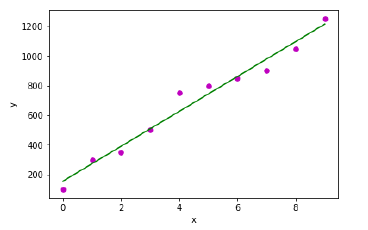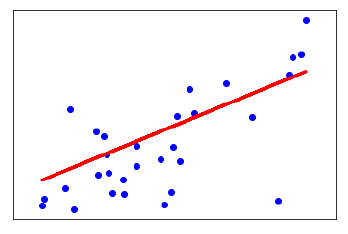# Machine Learning - Simple Linear Regression

It is the most basic version of linear regression which predicts a response using a single feature. The assumption in SLR is that the two variables are linearly related.

## Python Implementation

We can implement SLR in Python in two ways, one is to provide your own dataset and other is to use dataset from scikit-learn python library.

Example 1 − In the following Python implementation example, we are using our own dataset.

%matplotlib inline
import numpy as np
import matplotlib.pyplot as plt


Next, define a function which will calculate the important values for SLR −

def coef_estimation(x, y):


The following script line will give number of observations n −

n = np.size(x)


The mean of x and y vector can be calculated as follows −

m_x, m_y = np.mean(x), np.mean(y)


We can find cross-deviation and deviation about x as follows −

SS_xy = np.sum(y*x) - n*m_y*m_x
SS_xx = np.sum(x*x) - n*m_x*m_x


Next, regression coefficients i.e. b can be calculated as follows −

b_1 = SS_xy / SS_xx
b_0 = m_y - b_1*m_x
return(b_0, b_1)


Next, we need to define a function which will plot the regression line as well as will predict the response vector −

def plot_regression_line(x, y, b):


The following script line will plot the actual points as scatter plot −

plt.scatter(x, y, color = "m", marker = "o", s = 30)


The following script line will predict response vector −

y_pred = b + b*x


The following script lines will plot the regression line and will put the labels on them −

plt.plot(x, y_pred, color = "g")
plt.xlabel('x')
plt.ylabel('y')
plt.show()


At last, we need to define main() function for providing dataset and calling the function we defined above −

def main():
x = np.array([0, 1, 2, 3, 4, 5, 6, 7, 8, 9])
y = np.array([100, 300, 350, 500, 750, 800, 850, 900, 1050, 1250])

b = coef_estimation(x, y)
print("Estimated coefficients:\nb_0 = {} \nb_1 = {}".format(b, b))
plot_regression_line(x, y, b)
if __name__ == "__main__":
main()


Output

Estimated coefficients:
b_0 = 154.5454545454545
b_1 = 117.87878787878788Example 2 − In the following Python implementation example, we are using diabetes dataset from scikit-learn.

%matplotlib inline
import matplotlib.pyplot as plt
import numpy as np
from sklearn import datasets, linear_model
from sklearn.metrics import mean_squared_error, r2_score


Next, we will load the diabetes dataset and create its object −

diabetes = datasets.load_diabetes()


As we are implementing SLR, we will be using only one feature as follows −

X = diabetes.data[:, np.newaxis, 2]


Next, we need to split the data into training and testing sets as follows −

X_train = X[:-30]
X_test = X[-30:]


Next, we need to split the target into training and testing sets as follows −

y_train = diabetes.target[:-30]
y_test = diabetes.target[-30:]


Now, to train the model we need to create linear regression object as follows −

regr = linear_model.LinearRegression()


Next, train the model using the training sets as follows −

regr.fit(X_train, y_train)


Next, make predictions using the testing set as follows −

y_pred = regr.predict(X_test)


Next, we will be printing some coefficient like MSE, Variance score etc. as follows −

print('Coefficients: \n', regr.coef_)
print("Mean squared error: %.2f" % mean_squared_error(y_test, y_pred))
print('Variance score: %.2f' % r2_score(y_test, y_pred))


Now, plot the outputs as follows −

plt.scatter(X_test, y_test, color = 'blue')
plt.plot(X_test, y_pred, color = 'red', linewidth = 3)
plt.xticks(())
plt.yticks(())
plt.show()


Output

Coefficients:
[941.43097333]
Mean squared error: 3035.06
Variance score: 0.41machine_learning_with_python_regression_algorithms_linear_regression.htm